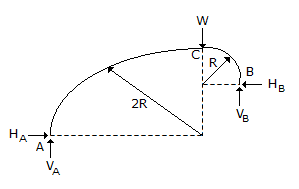# Civil Engineering - UPSC Civil Service Exam Questions - Discussion

5.

A three-hinged circular arch ACB is formed by two quadrants of circles AC and BC of radii 2R and R respectively with C as crown, as shown in the figure at side. Consider the following in respect of the reactive forces developed at support A and B due to concentrated load W at the Crown.
1. Line of action RA at A is inclined as 45° to the horizontal.
2. VA = VB = W/2
3. HB = 2 HA
4. HA = HB = W/2
Which of the above is/are correct ?[A]. 1 and 3 [B]. 2 and 3 [C]. 1, 2 and 4 [D]. 4 only

Explanation:

No answer description available for this question.

 Abc said: (Mar 13, 2019) Yes, option C is the correct answer.